# Definition

An `area chart` is really similar to a line chart and represents the evolution of a numeric variable. Basically, the X axis represents time or an ordered variable, and the Y axis gives the value of another variable. Data points are connected by straight line segments and the area between the x axis and the line is filled in with color or shading.

The following example shows the evolution of the bitcoin price between April 2013 and April 2018. Data comes from the CoinMarketCap website.

``````# Libraries
library(tidyverse)
library(hrbrthemes)
library(plotly)
library(patchwork)
library(babynames)
library(viridis)

data\$date <- as.Date(data\$date)

# plot
data %>%
ggplot( aes(x=date, y=value)) +
geom_area(fill="#69b3a2", alpha=0.5) +
geom_line(color="#69b3a2") +
ggtitle("Evolution of Bitcoin price") +
ylab("bitcoin price (\$)") +
theme_ipsum()``````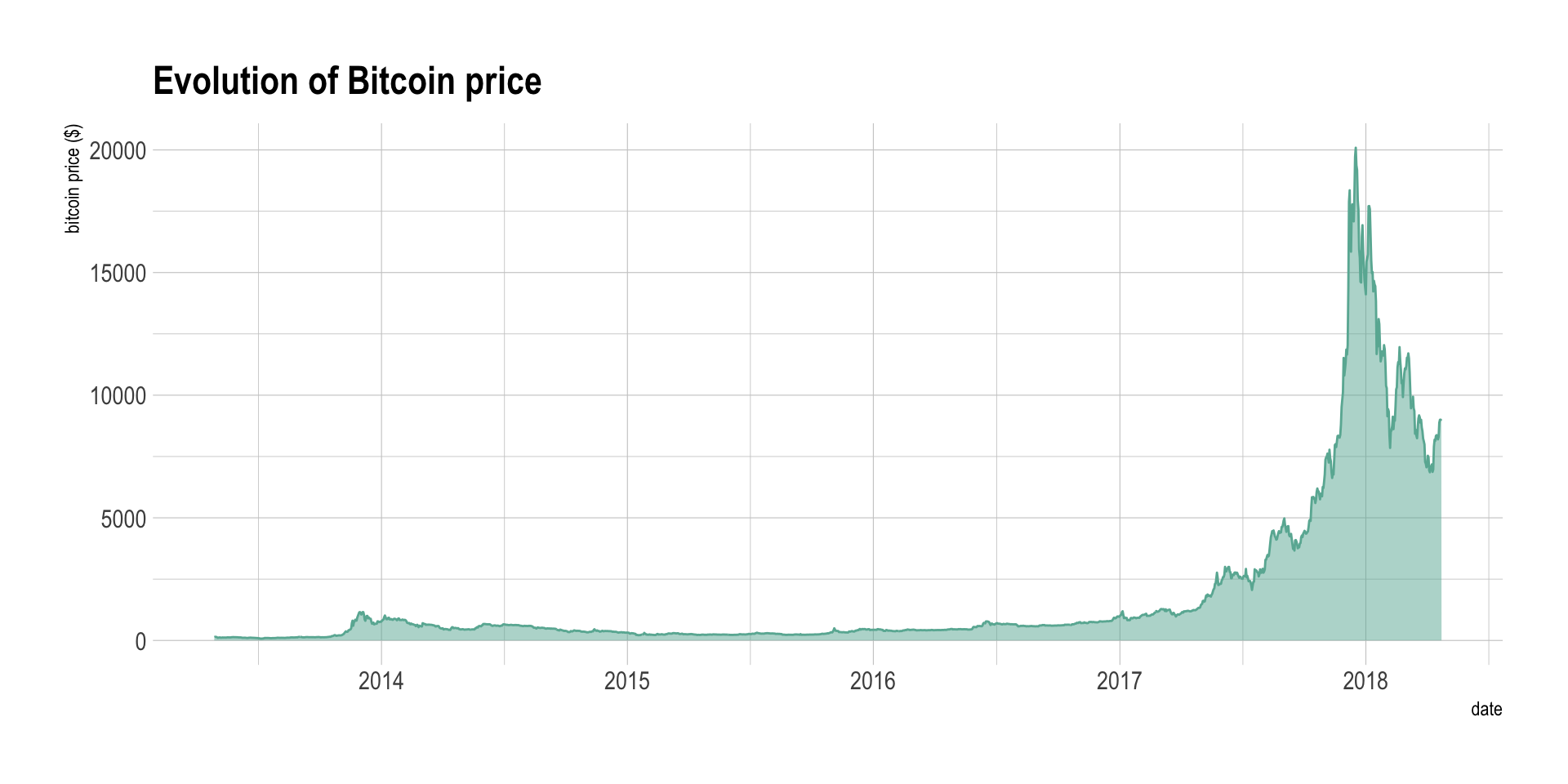# What for

Area chart is used to show the evolution of numeric variable. It is sometimes criticized for not optimizing the data-ink ratio, a data visualization principle that checks that no inks is used for nothing on the chart. Indeed, removing the area under the curve would make a line chart that conveys the same information. In my opinion area chart make a very good work to show an evolution and the filled area makes the pattern even more obvious.

Area chart can also be used to show the evolution of `several variables`. The most classic way is through a stacked area chart that is discussed in another section, but this can also be done using `small multiple`. Here is an example showing the evolution of a few baby name frequencies in the US between 1880 and 2015.

``````# Load dataset from github
don <- babynames %>%
filter(name %in% c("Ashley", "Amanda", "Mary", "Deborah",   "Dorothy", "Betty", "Helen", "Jennifer", "Shirley")) %>%
filter(sex=="F")

# Plot
don %>%
ggplot( aes(x=year, y=n, group=name, fill=name)) +
geom_area() +
scale_fill_viridis(discrete = TRUE) +
theme(legend.position="none") +
ggtitle("Popularity of American names in the previous 30 years") +
theme_ipsum() +
theme(
legend.position="none",
panel.spacing = unit(0, "lines"),
strip.text.x = element_text(size = 8),
plot.title = element_text(size=13)
) +
facet_wrap(~name, scale="free_y")``````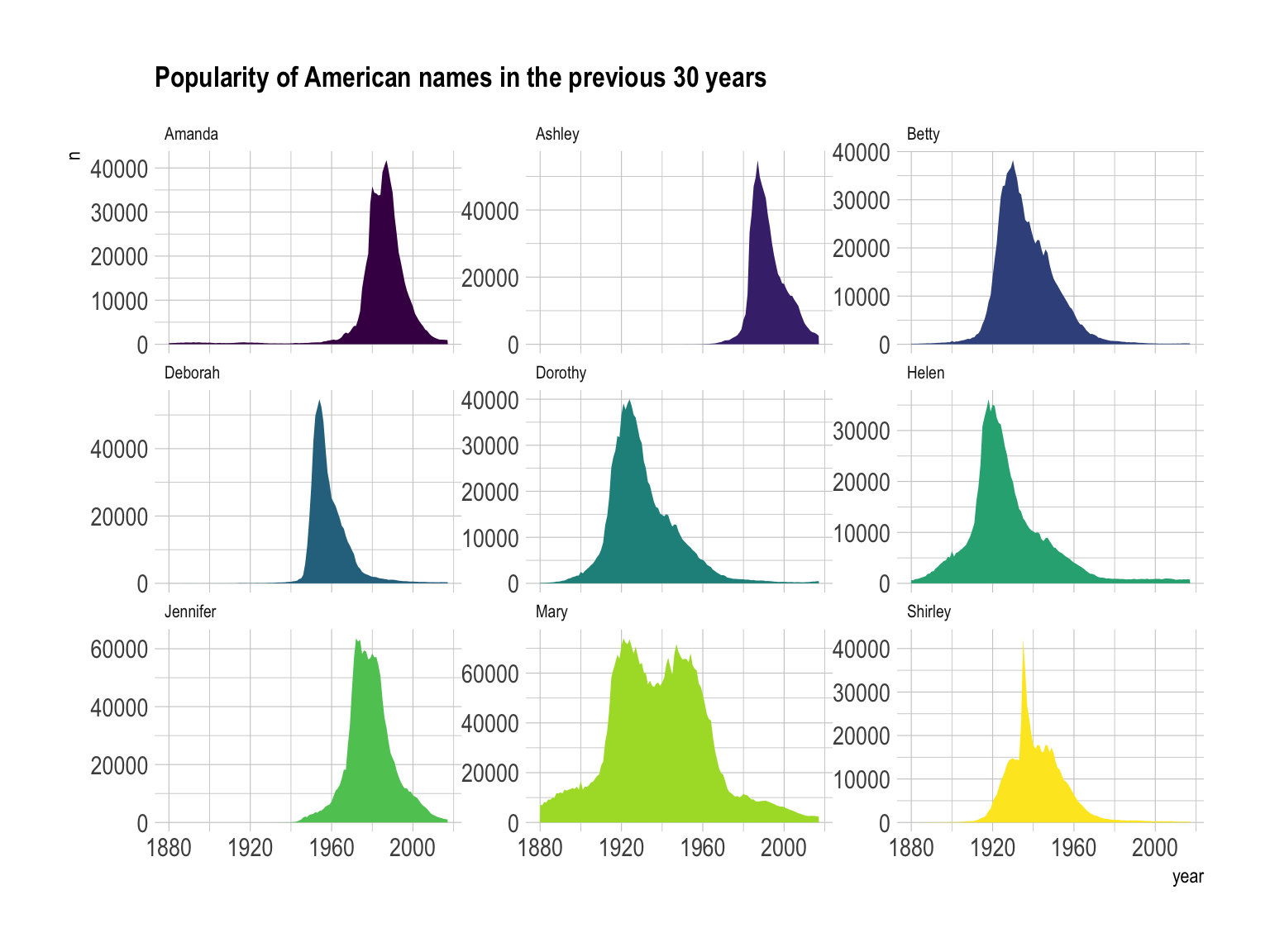Note: this graphic would deserve a discussion on the Y axis range to use and on the number of row & column to set. Watch this space.

# Variation

If the number of data points is low, it is advised to represent each individual observation with a dot. It allows to understand when exactly the observation have been made:

``````data %>%
tail(10) %>%
ggplot( aes(x=date, y=value)) +
geom_line(color="#69b3a2") +
geom_point(color="#69b3a2", size=4) +
ggtitle("Cuting") +
ylab("bitcoin price (\$)") +
theme_ipsum()``````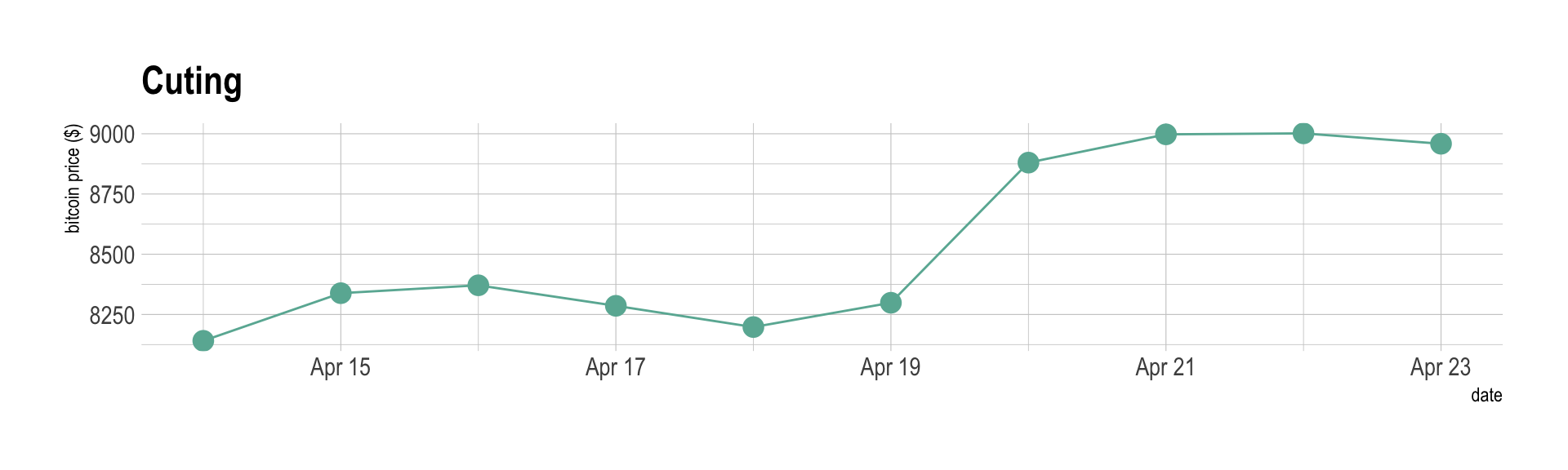Note that lines are also used to show trends in a scatterplot. Here is an example using Smoothed conditional means and showing confidence interval around it:

``````ggplot(mpg, aes(displ, hwy)) +
geom_point() +
geom_smooth(color="#69b3a2") +
theme_ipsum()``````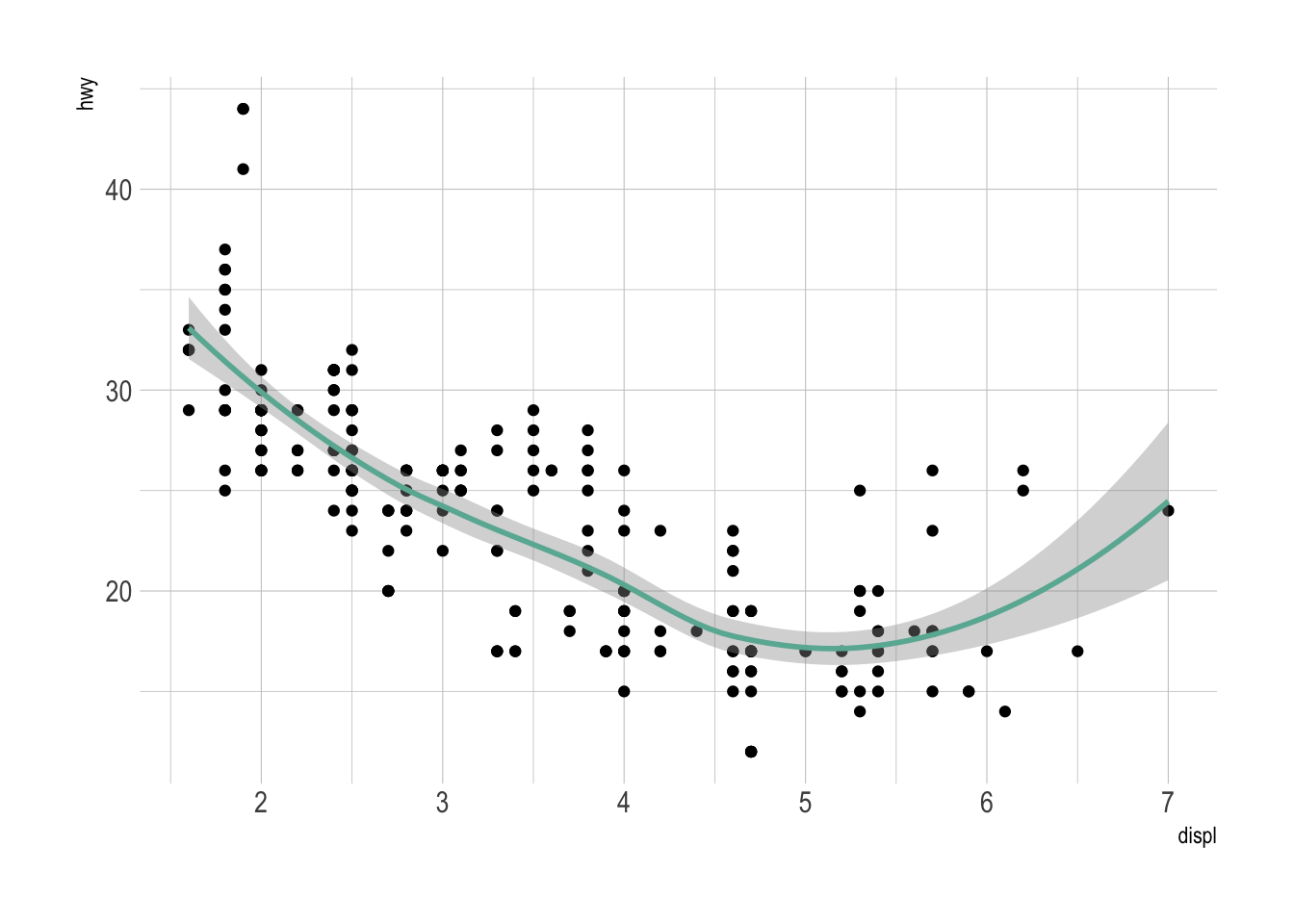Note: this example comes from the ggplot2 documentaion.

# Common caveats

The line chart is subject to a lot of discussion concerning potential caveats. Here is an overview:

• To cut or not to cut the Y axis? Wether or not the Y axis must start at 0 is a hot topic leading to intense debates. The graphic below presents the same data, starting at 0 (left) or not (right). Generally, line plot do not need to start at 0 since it allows to observe patterns more efficiently, but you probably want to learn more about it here.
``````p1 <- data %>%
tail(10) %>%
ggplot( aes(x=date, y=value)) +
geom_line(color="#69b3a2") +
geom_point(color="#69b3a2", size=4) +
ggtitle("Not cuting") +
ylab("bitcoin price (\$)") +
theme_ipsum() +
ylim(0,10000)

p2 <- data %>%
tail(10) %>%
ggplot( aes(x=date, y=value)) +
geom_line(color="#69b3a2") +
geom_point(color="#69b3a2", size=4) +
ggtitle("Cuting") +
ylab("bitcoin price (\$)") +
theme_ipsum()

p1 + p2``````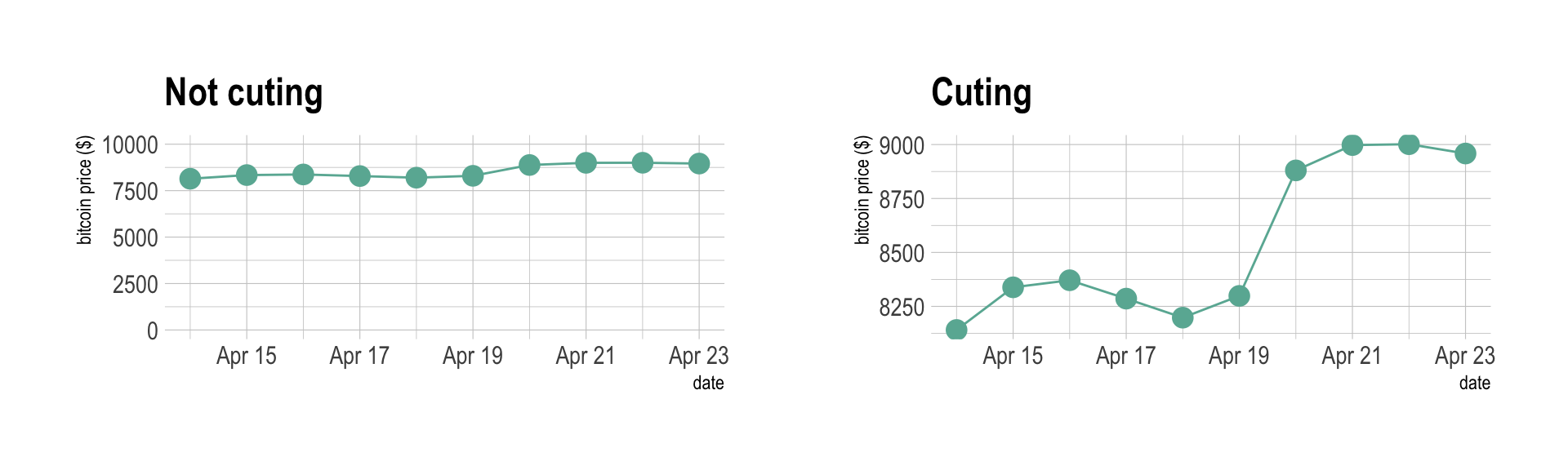• If you need to compare the evolution of 2 different variables, do not use dual axis. Indeed dual axis can show very different results depending on what range you apply to the axis. Read more about it.
• Mind the spaghetti chart: too many lines make the chart unreadable.
• Think about the aspect ratio of the graphic, extreme ratio make the chart unreadable.

# Related

The R and Python graph galleries are 2 websites providing hundreds of chart example, always providing the reproducible code. Click the button below to see how to build the chart you need with your favorite programing language.